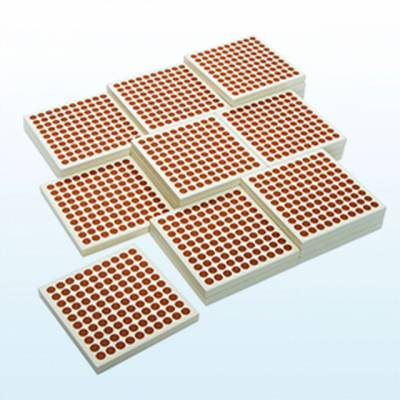CH - Mathematics
45 Wooden Hundred Squares
R 659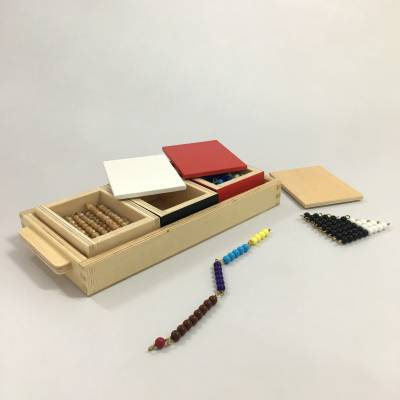CH - Mathematics
R 899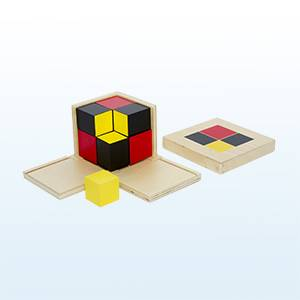CH - Mathematics
Algebraic Binomial Cube
R 899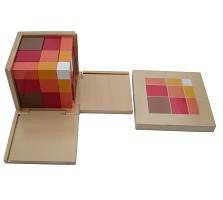CH - Mathematics
Arithmetic Trinomial Cube
R 999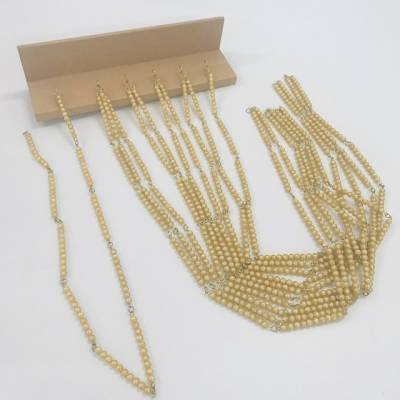CH - Mathematics
R 679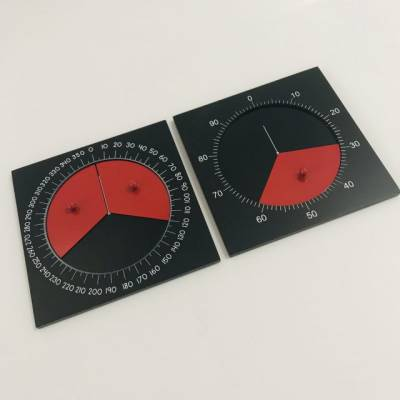CH - Mathematics
Centisimal Frame & Instrument for Measuring Angles
R 199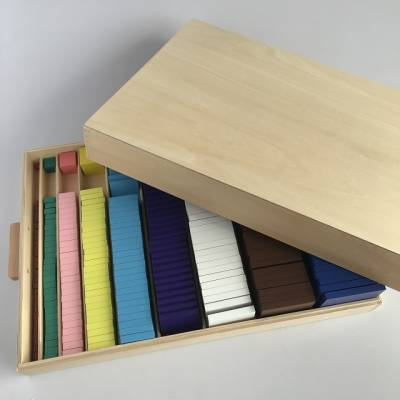CH - Mathematics
Cubing and Cube Root Material
R 4,399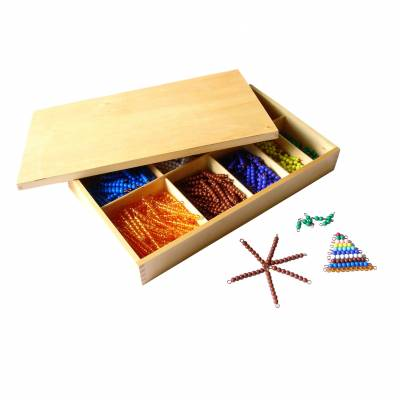CH - Mathematics